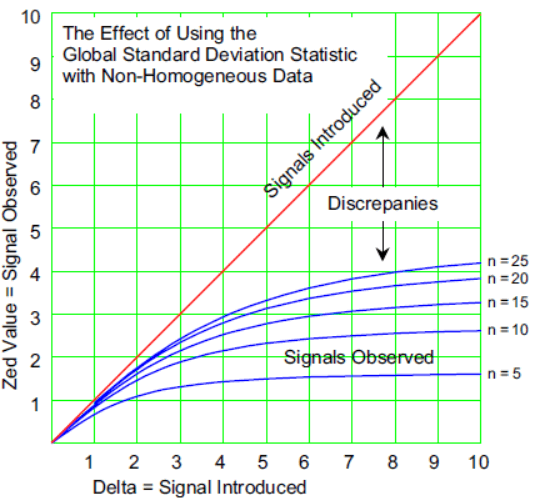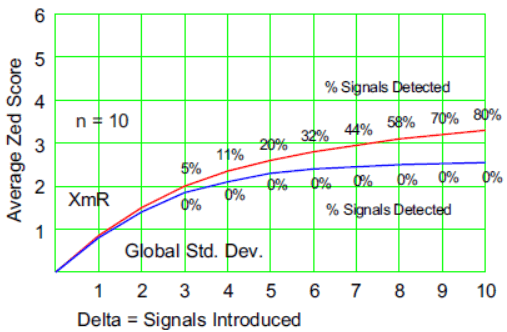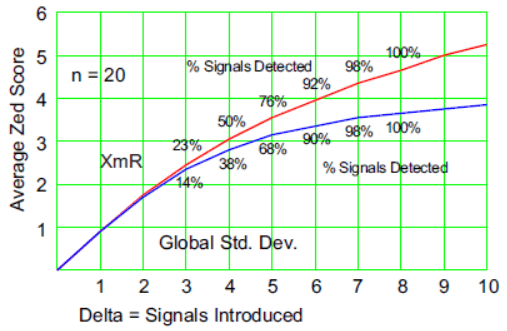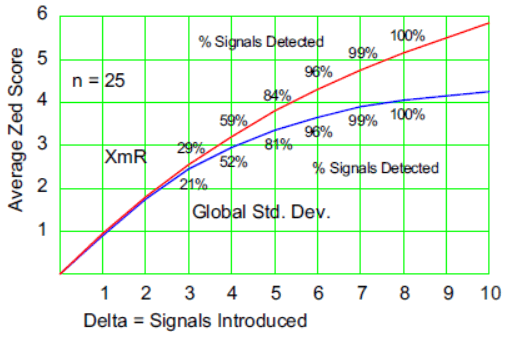#Quality Digest

Featured Product
This Week in Quality Digest Live
Statistics Features
Donald J. Wheeler
What do predictable processes have in common with chaos?
William A. Levinson
People can draw the wrong conclusions due to survivor, survey, and bad news bias.
Donald J. Wheeler
Does your approach do what you need?
Paul Laughlin
Correlation vs. causality
Donald J. Wheeler
In spite of what everyone says to the contrary
Statistics News
New capability delivers deeper productivity insights to help manufacturers meet labor challenges
Day and a half workshop to learn, retain, and transfer GD&T knowledge across an organization
Elsmar Cove is a leading forum for quality and standards compliance
InfinityQS’ quality solutions have helped cold food and beverage manufacturers around the world optimize quality and safety
User friendly graphical user interface makes the R-based statistical engine easily accessible to anyone
Collect measurements, visual defect information, simple Go/No-Go situations from any online device
Good quality is adding an average of 11 percent to organizations’ revenue growth
Ability to subscribe with single-user minimum, floating license, and no long-term commitment
A guide for practitioners and managersBio

Statistics

## The Global Standard Deviation Statistic

### Why it does not filter out the noise

Published: Monday, November 2, 2020 - 12:03

Every introductory class in statistics teaches how to obtain a global standard deviation statistic. While this descriptive statistic characterizes the dispersion of the data, it is not the correct value to use when looking for unusual values within the data. Since all of statistical inference is built around the search for unusual values, it is important to understand why we do not use the global standard deviation in this quest.

The descriptive statistics taught in introductory classes are appropriate summaries for homogeneous collections of data. But the real world has many ways of creating non-homogeneous data sets. Some of these involve unknown and unplanned changes in the process or procedure producing the measurements. When these occur we talk about “outliers” and “exceptional values.” Other ways of getting unusual values involve the deliberate manipulation of inputs for the purpose of creating changes in the observed data. Here we talk about detecting “signals” and obtaining “significant results.”

Regardless of what words we use, and regardless of whether the unusual values are intentional or accidental, the art of statistical analysis involves separating the potential signals from the probable noise. And this separation requires that we obtain some estimate of the noise level within our data to use as our filter. In pursuit of this estimate, the naive computation of the global standard deviation statistic is inappropriate.

To illustrate why this is so, we begin with a homogeneous data set consisting of n values. (Homogeneity is the term we use to describe data obtained by observing n independent and identically distributed random variables.) Let us further assume that these n original data have an average of 0.000 and a standard deviation statistic of 1.000. Further, to simplify the computations, let us assume that one of the n values is zero.

Now let us transform our homogenous data set into a non-homogeneous data set by replacing the zero value with some fixed value. Denote this fixed value by Delta. This change will shift the average of the modified data set from 0.000 to [ Delta / n ] and it will shift the global standard deviation statistic from 1.000 to:Consider the fixed value Delta as our signal. It is the unusual value that we will want to identify as the result of our analysis. To see how this introduced signal will show up within our modified data set, we use the standardization transformation. When we subtract the new average and divide by the global standard deviation statistic, and include the bias correction factor c4, we obtain the zed score for the value of Delta.When we use this formula with different values of Delta and n, we see how the inflation in the global standard deviation statistic created by introducing Delta actually reduces the standardized value for Delta. If we consider Delta as the signal we want to detect, the standardized value represents the signal we will actually observe. The discrepancies between the signals introduced and the signals observed may be seen in figure 1.Figure 1: How the global standard deviation hides signals

With n = 10, a 10 standard deviation shift will appear to be only 2.64 standard deviations above the average.

With n = 25, a 10 standard deviation shift will appear to be only 4.25 standard deviations above the average.

Thus, we see that when an unusual value is contained within our data set, that unusual value will inflate the global standard deviation statistic, which will in turn make the unusual value appear to be considerably smaller than it really is. As a result, any analysis technique that uses the global standard deviation statistic as the basis for separating the potential signals from the probable noise is going to be very insensitive. So what can be done?

### The foundation of modern statistical analysis

For the past 100 years, beginning with the two-sample Student’s t-test, the gold standard for filtering out the probable noise has been the use of within-subgroup measures of dispersion. In 1925 Sir Ronald Fisher built the analysis of variance on this foundation. In 1931 Walter Shewhart built process behavior charts on this foundation. Following John von Neumann’s introduction of the method of successive differences into the mainstream of mathematical techniques in 1941, W. J. Jennett was able to build the XmR chart on the foundation of within-subgroup variation. And in 1967 Ellis Ott built the analysis of means on the foundation of within-subgroup variation. So today all modern techniques of data analysis use the within-subgroup variation to filter out the probable noise as the basis for identifying any potential signals within the data.

To illustrate why this is the case, I performed a simple spreadsheet experiment. Using the standard normal distribution, I generated 10,000 data sets containing n = 10, 15, 20, and 25 values each. Then I added Delta to the second observation in each data set. Then I effectively placed each of these modified data sets on an XmR chart by computing the zed score for the second value in each data set. (Zed values greater than 3.0 correspond to points that would fall above the upper limit on the X chart.)Then, for these same modified data sets, I computed the zed score for the second values, using the global standard deviation statistic. (This is like using the global standard deviation to compute limits on the X chart.) Once again, zed scores greater than 3.0 correspond to the detection of the signal added.Figure 2 shows the results for n = 10. The curves show the averages of the 10,000 zed scores obtained for each of the different values of Delta. The percentages list how many times out of the 10,000 that the zed scores exceeded the cutoff of 3.0. (These percentages represent how many times the added signals were detected.)Figure 2: Moving ranges vs. standard deviations when n = 10

With n = 10 data, the XmR chart detected a 4 sigma signal 11 percent of the time, and it detected a 10 sigma signal 80 percent of the time. In contrast to this, not one of the 100,000 zed scores based on the global standard deviation statistic exceeded 3.0 regardless of the size of the signal introduced. Use the global standard deviation here, and you are guaranteed that you will find no signals.

Figure 3 shows the results for n = 15. As the data sets get larger the “contamination” introduced by the values for Delta decreases, and the average zed scores tend to get larger.Figure 3: Moving ranges vs. standard deviations when n = 15

Figure 4 shows the results for n = 20. The global standard deviation statistic lags behind simply because its computation effectively presumes that the data are completely homogeneous. When this presumption is wrong, the computation is misleadingFigure 4: Moving ranges vs. standard deviations when n = 20

Figure 5 shows the results for n = 25. Here, in spite of the substantial differences in the average zed-score curves, the percentages of detected signals are no longer so discrepant.Figure 5: Moving ranges vs. standard deviations when n = 25

When the data set and the signals get large enough, even the naive computations will detect the unusual value. However, using the within-subgroup dispersion (moving ranges, in this case) gives greater sensitivity throughout the range of different-sized signals and different-sized data sets. Since detecting the potential signals is the name of the game, this increased sensitivity is important in all types of data analysis.

Yet throughout the 20th century and continuing down to the present, various professional statisticians and engineers, writing in peer-reviewed journals, advocate the naive approach of using the global standard deviation in techniques for identifying unusual values within the data. Because of this continuing failure to appreciate the importance of the foundation of modern data analysis, next month’s column will review and compare several outlier detection techniques.

### Discuss